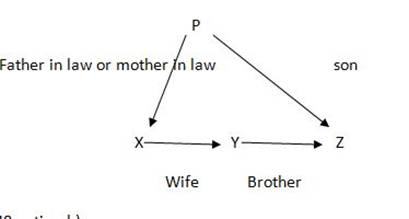## Blood Relation – Practice set 8

D.1-3) Study the following information carefully to answer the given questions

P @ Q means P is sister of Q

P % B means P is son of Q

P \$ Q means P is mother of Q

P # Q means P is father of Q

1. A @ I % D \$ F # G, what relationship can exist between D and G ?

a) Aunty

b) Grand Father

c) Mother

d) Grand Mother

e) None of these

d) Grand Mother

D

2. How is P related to O in the given expression ?

V \$ O # Y @ Z % P

a) Grandmother

b) Sister

c) Wife

d) Mother

e) None of these

c) Wife

C

3. Which of the following should come in place of ? to make the expression M is grandfather of S ?

U % M # R ? T # S

a) \$

b) %

c) @

d) #

e) None of these

c) @

C

4. Z is father of M. M is married to B. B is mother of T. B has only one daughter, T is married to O. R is son of O. How is B related to R ?

a) Mother

b) Father

c)Uncle

d)Grand mother

e) Grand father

d)Grand mother

D

5. K is brother of T. M is mother of K. W is brother of M. how is W related to T?

a) Maternal Uncle

b) Paternal Uncle

c) Grandfather

e) None of these

a) Maternal Uncle

A

6. P-Q means Q is son of P and P*Q means P is brother of Q, P/Q means Q is sister of P, and P+Q means P is mother of Q. Which of the following is definitely true about N*K-M/L?

a) K is father of L and M

b) L is the daughter of K and is the niece of her uncle N

c) K is the father of M and L-his son and daughter respectively

d) M is the uncle of K‘s brother N

e) None of these

b) L is the daughter of K and is the niece of her uncle N

B

7. If A+B means A is the sister of B; A-B means A is the brother of B; A*B means A is the daughter of B, which of the following shows the relation that E is the maternal uncle of D?

a) D+F*E

b) D-F*E

c) D*F+E

d) D*F-E

e) None of these

e) None of these

E

8. If A \$ B means “A is father of B‘ , A # B means ‘A is daughter of B‘ , A @ B means ‘A is sister of B‘, then how is K related to M in H @ K \$ L # M?

a) Husband

b) Uncle

c) Father

d) Cannot be determined

e) None of these

a) Husband

A

9. X’ is the wife of ‘Y’ and ‘Y’ is the brother of ‘Z’, ‘Z’ is the son of ‘P’. How is ‘P’ related to ‘x’?

a) Sister

b) Aunt

c) Brother

d) Uncle

d) Uncle

D

Explanation

Option (e)10. Pointing to a girl , Abhishek said , “she is daughter of the only child of my father .” How is Abhishek’s wife related to that girl?

a) Daughter

b) Mother

c) Aunt

d) Sister

e) Sister in law